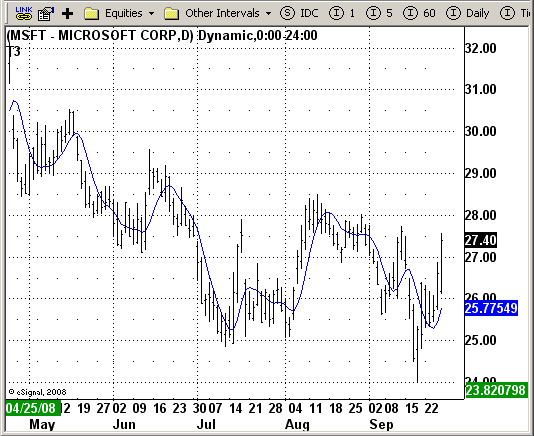# T3 Average

ICE Data Services -

T3Avg.efs
EFSLibrary - Discussion Board

File Name: T3Avg.efs

Description:
T3 Average

Formula Parameters:

• Length: 5
• Price Data To Use: Close

Notes:
This indicator plots the moving average described in the January, 1998 issue of S&C, p.57, "Smoothing Techniques for More Accurate Signals", by Tim Tillson. This indicator plots T3 moving average presented in Figure 4 in the article. T3 indicator is a moving average which is calculated according to formula:

`T3(n) = GD(GD(GD(n))), `

where GD - generalized DEMA (Double EMA) and calculating according to this:

`GD(n,v) = EMA(n) * (1+v)-EMA(EMA(n)) * v,`

where "v" is volume factor, which determines how hot the moving averageRs response to linear trends will be. The author advises to use v=0.7.
When v = 0, GD = EMA, and when v = 1, GD = DEMA. In between, GD is a less aggressive version of DEMA. By using a value for v less than1, trader cure the multiple DEMA overshoot problem but at the cost of accepting some additional phase delay.
In filter theory terminology, T3 is a six-pole nonlinear Kalman filter. Kalman filters are ones that use the error W in this case, (time series - EMA(n)) W to correct themselves. In the realm of technical analysis, these are called adaptive moving averages; they track the time series more aggres-sively when it is making large moves. Tim Tillson is a software project manager at Hewlett-Packard, with degrees in
mathematics and computer science. He has privately traded options and equities for 15 years.

T3Avg.efsEFS Code:

```/*********************************
Provided By:
eSignal (Copyright c eSignal), a division of Interactive Data
Formula Script (EFS) is for educational purposes only and may be
modified and saved under a new file name.  eSignal is not responsible
for the functionality once modified.  eSignal reserves the right
to modify and overwrite this EFS file with each new release.

Description:
T3 Average

Version:            1.0  09/24/2008

Notes:
This indicator plots the moving average described in the January, 1998 issue
of S&C, p.57, "Smoothing Techniques for More Accurate Signals", by Tim Tillson.
This indicator plots T3 moving average presented in Figure 4 in the article.
T3 indicator is a moving average which is calculated according to formula:
T3(n) = GD(GD(GD(n))),
where GD - generalized DEMA (Double EMA) and calculating according to this:
GD(n,v) = EMA(n) * (1+v)-EMA(EMA(n)) * v,
where "v" is volume factor, which determines how hot the moving average’s response
to linear trends will be. The author advises to use v=0.7.
When v = 0, GD = EMA, and when v = 1, GD = DEMA. In between, GD is a less aggressive
version of DEMA. By using a value for v less than1, trader cure the multiple DEMA
overshoot problem but at the cost of accepting some additional phase delay.
In filter theory terminology, T3 is a six-pole nonlinear Kalman filter. Kalman
filters are ones that use the error — in this case, (time series - EMA(n)) —
to correct themselves. In the realm of technical analysis, these are called adaptive
moving averages; they track the time series more aggres-sively when it is making large
moves. Tim Tillson is a software project manager at Hewlett-Packard, with degrees in
mathematics and computer science. He has privately traded options and equities for 15 years.

Formula Parameters:                     Default:
Length                                  5
Price Data To Use                      Close
**********************************/

var fpArray = new Array();
var bInit = false;

function preMain() {

setPriceStudy(true);
setStudyTitle("T3");
setCursorLabelName("Slope", 0);

setDefaultBarFgColor(Color.blue, 0);

var x=0;
fpArray[x] = new FunctionParameter("Length", FunctionParameter.NUMBER);
with(fpArray[x++]){
setLowerLimit(1);
setDefault(5);
}

fpArray[x] = new FunctionParameter("Price", FunctionParameter.STRING);
with(fpArray[x++]){
setName("Price Data To Use");
setDefault("close");
}
}

var xe1 = null;
var xe2 = null;
var xe3 = null;
var xe4 = null;
var xe5 = null;
var xe6 = null;
var xMyPrice = null;
var nT3Average = 0;

function main(Price, Length) {
var nState = getBarState();

if (nState == BARSTATE_ALLBARS) {
if (Price == null) Price = "close";
if (Length == null) Length = 5;
}

if ( bInit == false ) {
xMyPrice = eval(Price)();
xe1 = ema(Length, xMyPrice);
xe2 = ema(Length, xe1);
xe3 = ema(Length, xe2);
xe4 = ema(Length, xe3);
xe5 = ema(Length, xe4);
xe6 = ema(Length, xe5);
bInit = true;
}

if (xe1.getValue(0) == null || xe2.getValue(0) == null || xe3.getValue(0) == null ||
xe4.getValue(0) == null || xe5.getValue(0) == null || xe6.getValue(0) == null) return;

var b = 0.7;
var c1 = -b*b*b;
var c2 = 3*b*b+3*b*b*b;
var c3 = -6*b*b-3*b-3*b*b*b;
var c4 = 1+3*b+b*b*b+3*b*b;

nT3Average = c1 * xe6.getValue(0) + c2 * xe5.getValue(0) + c3 * xe4.getValue(0) + c4 * xe3.getValue(0);

return nT3Average;
}
```